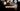# Leetcode | Solution of Reverse Vowels of a String in JavaScript

May 12th, 2020
|

In this post, we will solve Reverse Vowels of a String from leetcode and compute it's time and space complexities. Let's begin.

# Problem Statement

The question can be found at leetcode Reverse Vowels of a String problem.

The problem states that we are given a string and we need to reverse the vowels in the string. The question is very much similar to the reverse string problem we discussed.

# Solution

The question doesn't specify anything about the case. So we need to keep that in mind. The approach is as follows

• We declare an array of vowels and assign all the uppercase and lowercase vowels to it
• Next, we convert the string to an array and apply two pointer approach to reverse the vowels
• The only thing to note that while reversing, we need to check if strings at left and right indices are vowels.
• To check for vowels we can just see if both the strings are present in our vowels array
• Once we find two vowels, we swap it
• when the loop exits, we have an array with vowels reversed, we join it back and return it.

I have discussed two pointer approach in reverse string problem, so please check that for more details.

We have discussed the approach, I urge you to go ahead on leetcode and give it another try.If you are here, it means something went wrong in implementation or you are just too lazy. In any case, let's see a simple implementation of the above logic.
``````var reverseVowels = function (s) {
const arr = s.split("");

let left = 0, right = arr.length;

const vowels = ['A', 'E', 'I', 'O', 'U', 'a', 'e', 'i', 'o', 'u']

while (left < right) {
if (vowels.indexOf(arr[left]) === -1) {
left++;
continue;
}
if (vowels.indexOf(arr[right]) === -1) {
right--;
continue;
}

const temp = arr[left];
arr[left] = arr[right];
arr[right] = temp;

left++;
right--;
}

return arr.join("")
};``````

As discussed, we convert the string to an array, declare a vowel array and declare two variables to point to the left and right end of the string. We iterate over the string and check if the characters at both indices are vowels. In case it is, we swap them and move on with the loop.

At last, we join back the array and return it.

Here are the stats on submission

``````Status: Accepted
Runtime: 68ms
Memory: 38.5MB``````

## Time and space complexity

### Time complexity

We are looping over the string array once, so time complexity would be O(n).

### Space complexity

We are using extra space to store the string array. So space complexity is linear, O(n).

# Summary

So, we solved the Reverse Vowels of a String problem by using two pointer method and calculated the time and space complexities.

I hope you enjoyed solving this question. This is it for this one, complete source code for this post can be found on my Github Repo. Will see you in the next one.